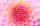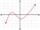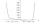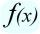# Eq2 2

Solve following equation with quadratic members and rational function:

(x2+1)/(x-4) + (x2-1)/(x+3) = 23

Result

x1 =  -2.733
x2 =  5.786
x3 =  8.947

#### Solution:Leave us a comment of example and its solution (i.e. if it is still somewhat unclear...):

Showing 1 comment:#### To solve this verbal math problem are needed these knowledge from mathematics:

Looking for help with calculating roots of a quadratic equation? Do you have a linear equation or system of equations and looking for its solution? Or do you have quadratic equation?

## Next similar examples:

1. Reciprocal equation 2Solve this equation: x + 5/x - 6 = 4/11
2. Solve 3Solve quadratic equation: (6n+1) (4n-1) = 3n2
3. Variation equationSolve combinatorics equation: V(2, x+8)=72
4. Quadratic function 2Which of the points belong function f:y= 2x2- 3x + 1 : A(-2, 15) B (3,10) C (1,4)
5. EquationEequation f(x) = 0 has roots x1 = 64, x2 = 100, x3 = 25, x4 = 49. How many roots have equation f(x2) = 0 ?
6. VariableFind variable P: PP plus P x P plus P = 160
7. DiscriminantDetermine the discriminant of the equation: ?
8. EquationEquation ? has one root x1 = 8. Determine the coefficient b and the second root x2.
9. RootsDetermine the quadratic equation absolute coefficient q, that the equation has a real double root and the root x calculate: ?
10. Quadratic equationFind the roots of the quadratic equation: 3x2-4x + (-4) = 0.
11. Quadratic equationQuadratic equation ? has roots x1 = -26 and x2 = -86. Calculate the coefficients b and c.
12. Evaluation of expressionsIf a2-3a+1=0, find (i)a2+1/a2 (ii) a3+1/a3
13. Square root 2If the square root of 3m2 +22 and -x = 0, and x=7, what is m?
14. AsymptoteWhat is the vertical asymptote of ?
15. Unknown numberI think number. If subtract from the twelfth square the ninth square I get a number 27 times greater than the intended number. What is this unknown number?
16. DerivationExists a function whose derivation is the same function?
17. Theorem proveWe want to prove the sentence: If the natural number n is divisible by six, then n is divisible by three. From what assumption we started?